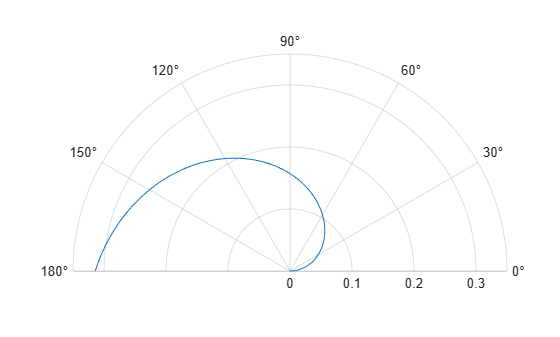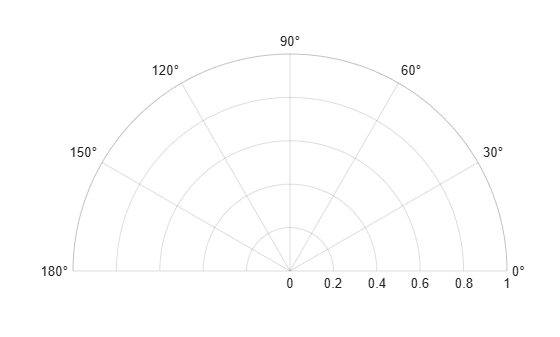# thetalim

Set or query theta-axis limits for polar axes

## Syntax

``thetalim(limits)``
``thetalim(limitmode)``
``tl = thetalim``
``m = thetalim("mode")``
``___ = thetalim(pax,___)``

## Description

### Specify Limits

example

````thetalim(limits)` specifies the theta-axis limits for the current polar axes. Specify `limits` as a two-element vector of the form `[thetamin thetamax]`, where `thetamax` is a numeric value greater than `thetamin`.```

example

````thetalim(limitmode)` specifies automatic or manual limit selection. The `limitmode` can have either of two values: `"auto"` — Enable automatic limit selection. MATLAB® chooses the theta-axis limits.`"manual"` — Freeze the theta-axis limits at their current value. ```

### Query Limits

````tl = thetalim` returns a two-element vector containing the limits for the current polar axes.```
````m = thetalim("mode")` returns the current value of the limits mode, which is either `'auto'` or `'manual'`. By default, the mode is automatic unless you specify limits or set the mode to manual.```

### Specify Target Axes

example

````___ = thetalim(pax,___)` uses the polar axes object specified by `pax` instead of the current polar axes. Specify `pax` as the first input argument. Include additional input or output arguments only if the original syntax supported them.```

## Examples

collapse all

Create a polar plot and change the theta-axis limits.

```theta = linspace(0,pi); rho = theta/10; polarplot(theta,rho) thetalim([0 180])```Set the limits back to the original values.

`thetalim('auto')`Set the limits for a specific polar axes by specifying the polar axes object as the first input to `thetalim`. Otherwise, `thetalim` sets the limits for the current axes.

```pax = polaraxes; thetalim(pax,[0 180])```## Input Arguments

collapse all

Minimum and maximum limits, specified as a two-element vector of the form ```[thetamin thetamax]```. When you specify the limits, the `ThetaLim` property for the polar axes object updates to the specified values.

Example: `[0 1]`

Limit mode, specified as one of the following values:

• `"auto"` — Enable automatic limit selection, which is based on the total span of the data. MATLAB chooses the theta-axis limits.

• `"manual"` — Freeze the limits at the current values.

When you specify this argument, MATLAB sets the `ThetaLimMode` property of the axes to the value you specify. However, the `ThetaLimMode` property changes to `"manual"` whenever you set the theta-axis limits explicitly, either by calling `thetalim(limits)`, or by setting the value of the `ThetaLim` property on the axes.

Polar axes, or an array of polar axes. If you do not specify this argument, then `thetalim` modifies the current axes (provided that the current axes is a polar axes object).

## Output Arguments

collapse all

Current limits, returned as a two-element vector of the form ```[thetamin thetamax]```. Querying the limits returns the value of the `ThetaLim` property for the polar axes object.

Current limits mode, returned as one of these values:

• `'auto'` — Limits automatically update to reflect changes in the data.

• `'manual'` — Limits do not automatically update.

Querying the theta-axis limits mode returns the value of the `ThetaLimMode` property for the polar axes object.

## Version History

Introduced in R2016a